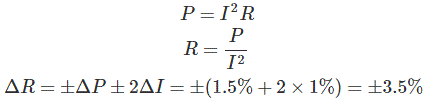# MCQs on Electrical And Electronics Measurements

##### Page 15 of 19. Go to page 1 2 3 4 5 6 7 8 9 10 11 12 13 14 15 16 17 18 19
01․ Schering bridge is used to measure which of the following?
Frequency.
Inductance.
Capacitance.
Resistance.

Wien bridge is used to measure the frequency. Maxwell bridge and Anderson bridge are used to find the inductance. Schering bridge is used to measure the capacitance and Whetstone bridge and Kelvin bridge are used to find the resistance.

02․ A moving coil of a meter has 100 turns, and a length and depth of 10 mm and 20 mm respectively. It is positioned in a uniform radial flux density of 200 mT. The coil carries a current of 50 mA. The torque on the coil is
200 µNm.
100 µNm.
2 µNm.
1 µNm.

Torque on the coil T = NBAI Where N = 100, B = 200 mT, A = Area of the coil = length X breadth = 200 X 10-6 sq. mt., I = current through the coil = 50 mA . ⇒ T = 200 µNm.

03․ Attraction and repulsion type instruments are the two different types of which instrument ?
Moving iron type.
Multipliers.
Dynamo-meter type.
Induction type.

The attraction and repulsion type instruments are called as moving iron instruments because the soft iron piece is placed in a magnetic field to become strongly magnetized. Hence, due to the attraction or repulsion action happens in the iron piece and thereby it moves. That is why those instruments are also called as moving iron instruments.

04․ A thermometer having first order dynamics is subjected to a sudden temperature change of 30oc to 150oc. If it has he time constant of 5 sec, what temperature will be indicated by after 5 sec?
150o.
105.6o.
63.25o.
30o.

For first order system, output c(t) = k0 + (ki + k0)(e-(t/τ)) Where, ki = Initial temperature; k0 = Final temperature; c(t) = 150 + (30 - 150) e-(5/5); c(t) = 150 - (120 × 0.37); c(t) = 105.6 oc.

05․ Following is a 16-bit register for 8085 microprocessor
Stack pointer
Accumulator
Register B
Register C

In a 8085 microprocessor Accumulator, register B & C are of 8-bit whereas stack pointer is a 16-bit register.

06․ A 8085 microprocessor consist of
Arithmetic and Logic Units (ALU), Control Unit (CU), Registers and Memory.
Arithmetic and Logic Units (ALU), Control Unit (CU) and Registers.
Arithmetic and Logic Units (ALU), Control Unit (CU), Registers and I/O devices.
Arithmetic and Logic Units (ALU), Control Unit (CU) and I/O devices.

A 8085 microprocessor consist of Arithmetic and Logic Units (ALU), Control Unit (CU) and Registers. It does not have memory inside it. We have to connect memory externally.

07․ A voltmeter has 100 scale divisions and can measure up to 100 V. Each division can be read to ½ division. The resolution of the voltmeter is
1 V
0.5 V
1.5 V
2 V

Resolution is nothing but smallest change in the input that can be measured by the instrument. In the given instrument smallest change in the input that can be measured is ½ division. 100division = 100volt 1division = 1volt 1/2division = 0.5volt

08․ The resistance of a circuit is found by measuring current flowing and the power fed into the circuit. If the limiting errors in the measurement of power and current are ± 1.5% and ± 1.0% respectively, the limiting error in the measurement of resistance
± 3.5%
± 1%
± 2.5%
± 1.5%

We know power09․ The difference between the measured value and the true value is called
probable error
absolute error
gross error
relative error

The difference between the measured value and the true value is called absolute error.

10․ The errors introduced by an instrument fall in which category ?
Systematic errors
Random errors
Gross errors
Environmental errors

Systematic errors may be due to instrumental error.

<<<1314151617>>>